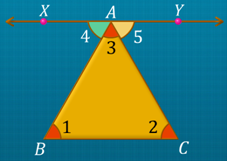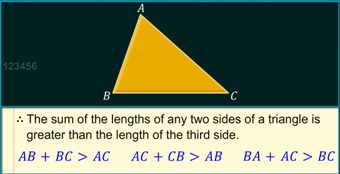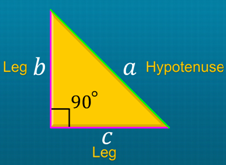Notes On Properties of Triangles - CBSE Class 7 Maths
Angle sum property The sum of the three angles of a triangle is 180°. e.g. If A, B and C are the angles of a triangle ABC, then ∠A + ∠B + ∠C = 180°. Proof: Consider a triangle ABC. Let line XY be parallel to side BC at A. AB is a transversal that cuts the line XY and AB, at A and B, respectively.  As the alternate interior angles are equal, ∠1 = ∠4 and ∠2 = ∠5.   ∠4, ∠3 and ∠5 form linear angles, and their sum is equal to 180°. ⇒ ∠4 + ∠3 + ∠5 = 180° ⇒ ∠1 + ∠2 + ∠3 = 180° Hence, the sum of the three angles of a triangle is 180°.Exterior angle property An exterior angle of a triangle is equal to the sum of its opposite interior angles. e.g. If ∠4 is an exterior angle of ΔABC,  ∠1 and ∠2 are the interior opposite angles, then ∠4 = ∠1 + ∠2. The sum of the lengths of any two sides of a triangle is greater than the third side.In a right-angled triangle, the side opposite the right angle is called the hypotenuse, and the other two sides are called its legs. Pythagorean theorem In a right-angled triangle, the square of the hypotenuse is equal to the sum of the squares of the other two sides.If b and c are legs and a is the hypotenuse of a right angled triangle then, a2 = b2 + c2. Converse of Pythagorean theorem If the sum of the squares on two sides of a triangle is equal to the square of the third side, then the triangle must be a right-angled triangle.

#### Summary

Angle sum property The sum of the three angles of a triangle is 180°. e.g. If A, B and C are the angles of a triangle ABC, then ∠A + ∠B + ∠C = 180°. Proof: Consider a triangle ABC. Let line XY be parallel to side BC at A. AB is a transversal that cuts the line XY and AB, at A and B, respectively.  As the alternate interior angles are equal, ∠1 = ∠4 and ∠2 = ∠5.   ∠4, ∠3 and ∠5 form linear angles, and their sum is equal to 180°. ⇒ ∠4 + ∠3 + ∠5 = 180° ⇒ ∠1 + ∠2 + ∠3 = 180° Hence, the sum of the three angles of a triangle is 180°.Exterior angle property An exterior angle of a triangle is equal to the sum of its opposite interior angles. e.g. If ∠4 is an exterior angle of ΔABC,  ∠1 and ∠2 are the interior opposite angles, then ∠4 = ∠1 + ∠2. The sum of the lengths of any two sides of a triangle is greater than the third side.In a right-angled triangle, the side opposite the right angle is called the hypotenuse, and the other two sides are called its legs. Pythagorean theorem In a right-angled triangle, the square of the hypotenuse is equal to the sum of the squares of the other two sides.If b and c are legs and a is the hypotenuse of a right angled triangle then, a2 = b2 + c2. Converse of Pythagorean theorem If the sum of the squares on two sides of a triangle is equal to the square of the third side, then the triangle must be a right-angled triangle.

Next